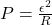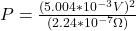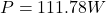## A length of 20-gauge copper wire (of diameter 0.8118 mm) is formed into a circular loop with a radius of 15.0 cm. A magnetic field perpendic

Question

A length of 20-gauge copper wire (of diameter 0.8118 mm) is formed into a circular loop with a radius of 15.0 cm. A magnetic field perpendicular to the plane of the loop increases from zero to 17.0 mT in 0.24 s. Find the average electrical power dissipated in the process.

in progress 0
2 weeks 2021-08-29T18:42:58+00:00 1 Answers 0 views 0

1. To solve this problem we will first find the value of the electric potential on the body. To find it previously we will find all the variables on which it depends, between them is the magnetic field and the Area. Once calculated we will proceed to calculate the resistance of the cable to finally find the Power.

The induced emf in a loop is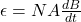The average rate of change of magnetic field is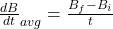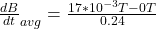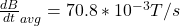The cross sectional area of the loop is geometrically as,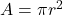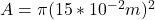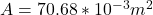The number of turns in the loop N=1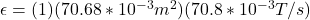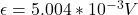The length of the copper wire is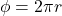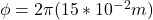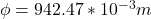With this value we can now calculate the resistance of the copper wire, then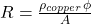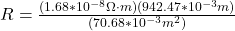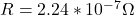Finally the power dissipated in the wire is given by the relation between the electric potential and the Resistance, then